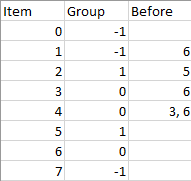# Sort Items by Groups Respecting DependenciesThere are n items each belonging to zero or one of m groups where group[i] is the group that the i-th item belongs to and it’s equal to -1 if the i-th item belongs to no group. The items and the groups are zero indexed. A group can have no item belonging to it.

Return a sorted list of the items such that:

• The items that belong to the same group are next to each other in the sorted list.
• There are some relations between these items where beforeItems[i] is a list containing all the items that should come before the i-th item in the sorted array (to the left of the i-th item).

Return any solution if there is more than one solution and return an empty list if there is no solution.

Example 1:Input: n = 8, m = 2, group = [-1,-1,1,0,0,1,0,-1], beforeItems = [[],,,,[3,6],[],[],[]]
Output: [6,3,4,1,5,2,0,7]


Example 2:

Input: n = 8, m = 2, group = [-1,-1,1,0,0,1,0,-1], beforeItems = [[],,,,,[],,[]]
Output: []
Explanation: This is the same as example 1 except that 4 needs to be before 6 in the sorted list.


Constraints:

• 1 <= m <= n <= 3*10^4
• group.length == beforeItems.length == n
• -1 <= group[i] <= m-1
• 0 <= beforeItems[i].length <= n-1
• 0 <= beforeItems[i][j] <= n-1
• i != beforeItems[i][j]
• beforeItems[i] does not contain duplicates elements.

Sol:

Two topological sorts: one for the groups and one for elements inside a group.

Note: my union find code is unnecessary because the group is GIVEN by the prompt!

I’m making things too complicated!

class Solution {
public:
int find_root(int x, vector<int>& root){
if(root[x] == x) return x;
return root[x] = find_root(root[x], root);
}

void union_two(int x, int y, vector<int>& root, vector<int>& rank, vector<vector<int>>& cld){
int xx = find_root(x, root);
int yy = find_root(y, root);
if(rank[xx] > rank[yy]) swap(x,y);
if(rank[xx] == rank[yy]) rank[yy]++;
root[xx] = yy;
cld[yy].insert(cld[yy].end(), cld[xx].begin(), cld[xx].end());
}

vector<int> topo_sort(vector<int>& v, vector<vector<int>>& cld, vector<vector<int>>&edge, vector<int>& deg,
vector<vector<int>>&node_edge, vector<int>& node_deg, int status){
if(v.size()==1) return v;
queue<int> q;
vector<int> ans;
for(int i =0;i<v.size();i++){
if(node_deg[v[i]] == 0) q.push(v[i]);
}
while(!q.empty()){
int x = q.front();q.pop();
cout<<x<<"\n";

if(status == 0){
vector<int> vx = topo_sort(cld[x], cld, edge, deg, edge, deg,1);
ans.insert(ans.end(), vx.begin(), vx.end());
}else{
ans.push_back(x);
}
for(int y: node_edge[x]){
node_deg[y]--;
if(node_deg[y] == 0) q.push(y);
}
}
return ans;
}

vector<int> sortItems(int n, int m, vector<int>& group, vector<vector<int>>& beforeItems) {
vector<int> grp(m, -1);
vector<int> root(n);
vector<int> rank(n,1);
vector<vector<int>> cld(n);
vector<vector<int>> edge(n);
vector<int> deg(n,0);

vector<vector<int>> node_edge(n);
vector<int> node_deg(n,0);

for(int i=0; i<n; i++){
root[i] = i;
cld[i].push_back(i);

int g = group[i];
if(g==-1) continue;
if(grp[g] != -1){
union_two(i, grp[g], root, rank, cld);
}else{
grp[g] = i;
}
}
for(int i=0; i<n; i++){
for(int j : beforeItems[i]){
if(find_root(i, root) == find_root(j, root)){
edge[j].push_back(i);
deg[i]++;
}
else{
node_edge[root[j]].push_back(root[i]);
node_deg[root[i]]++;
}
}
}
set<int> roots;
vector<int> allofthem;
for(int i=0; i<n; i++){
roots.insert(find_root(i, root));
// cout<<deg[i]<<" ";
}

for(int i: roots){
allofthem.push_back(i);
}
vector<int> v = topo_sort(allofthem, cld, edge, deg, node_edge, node_deg,0);
if(v.size()==n){
return v;
}else{
return vector<int>();
}

}
};